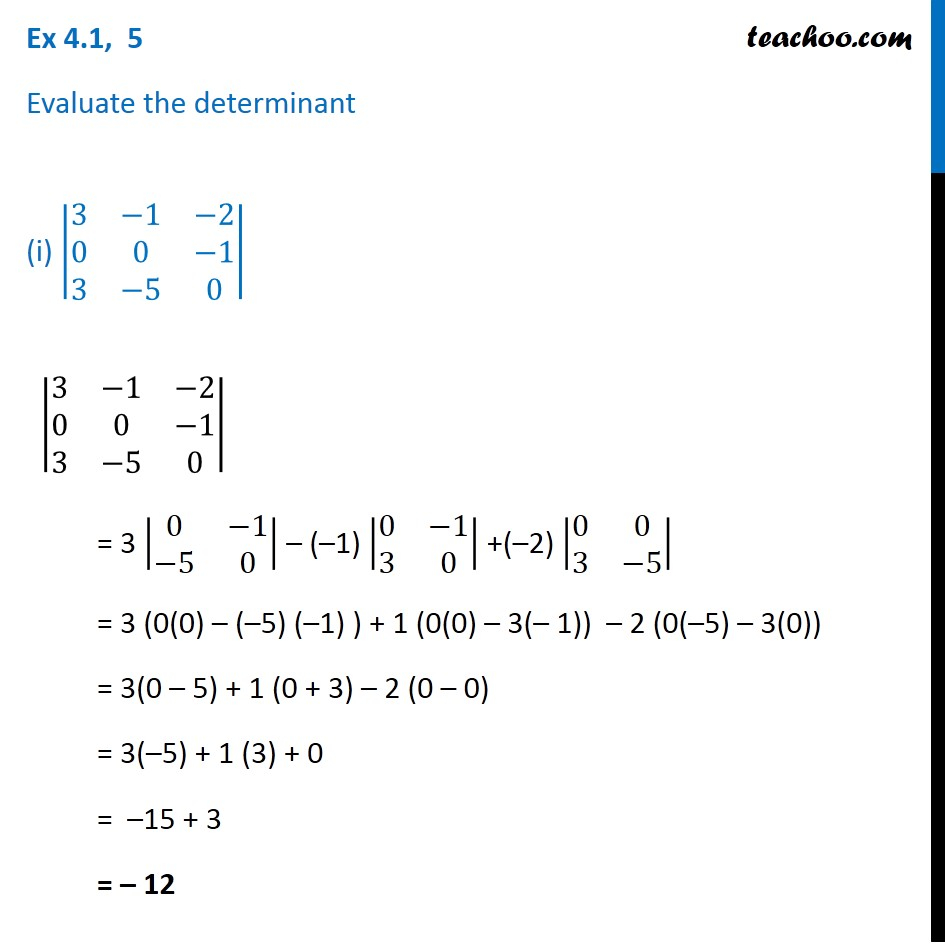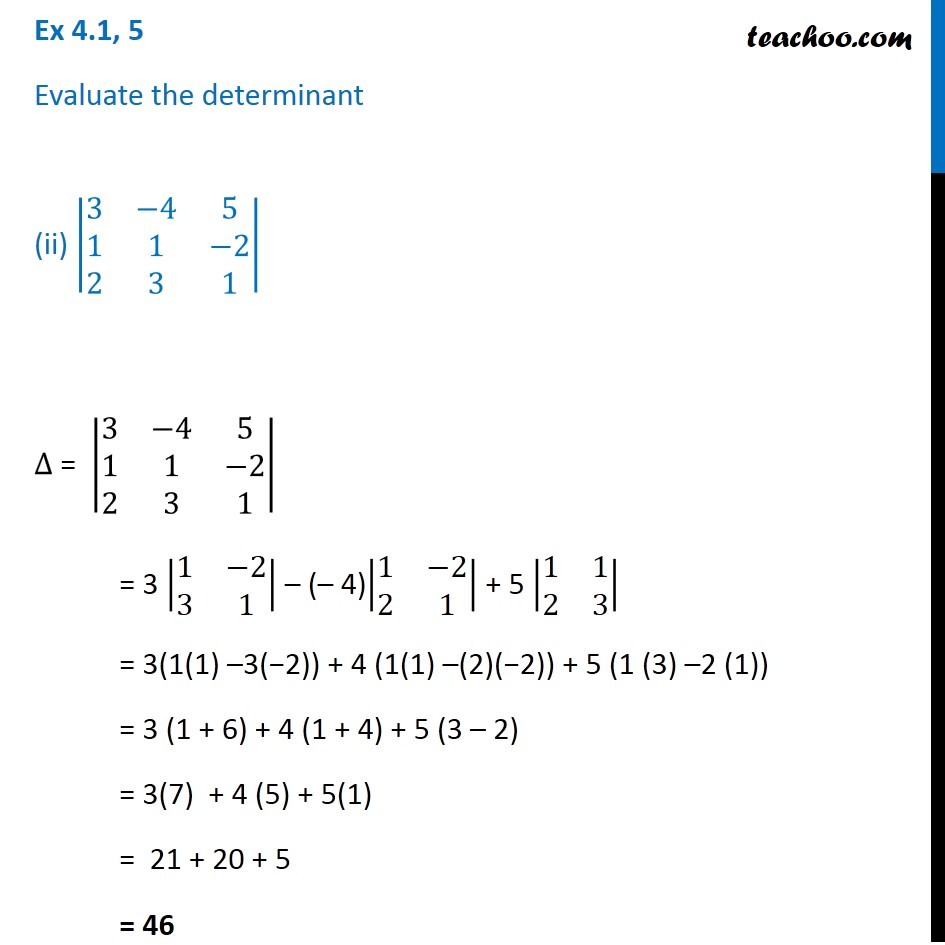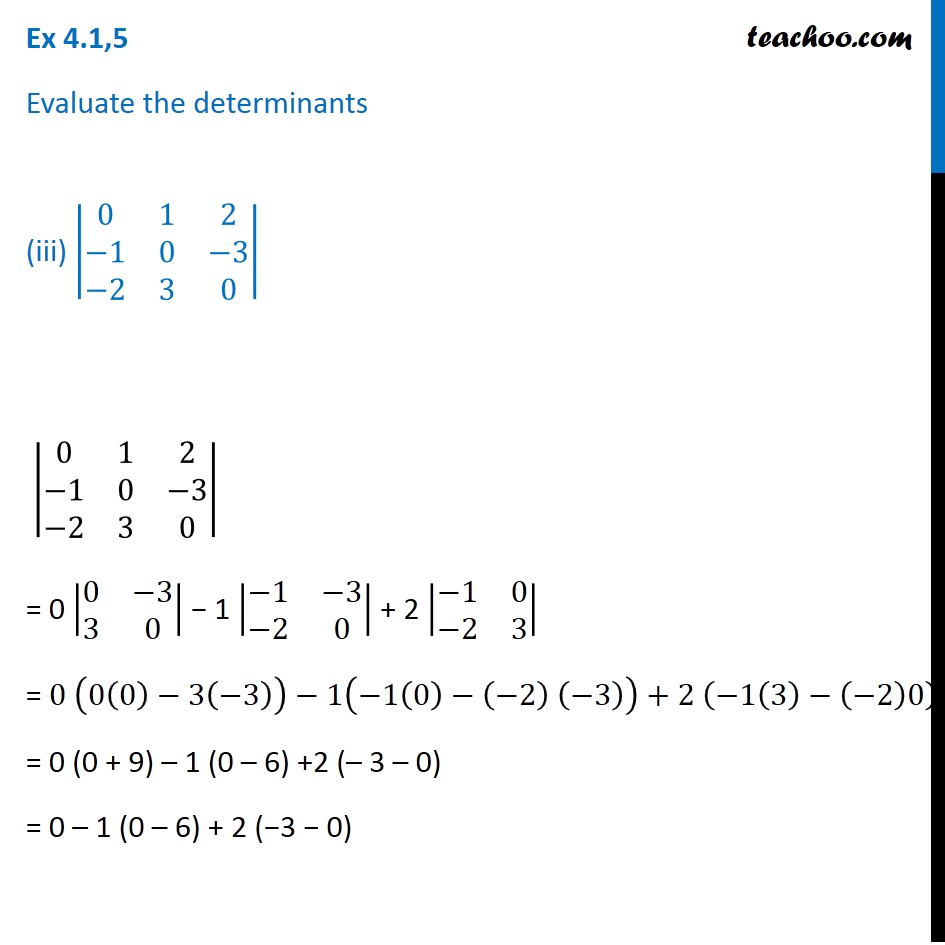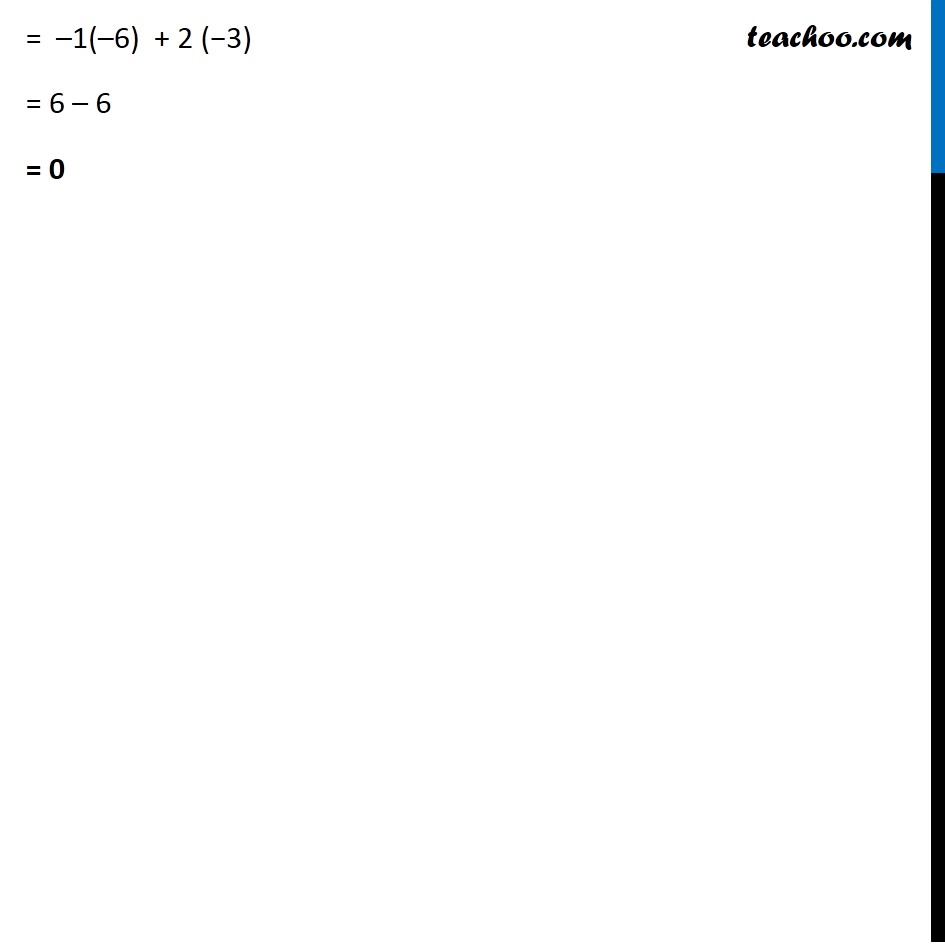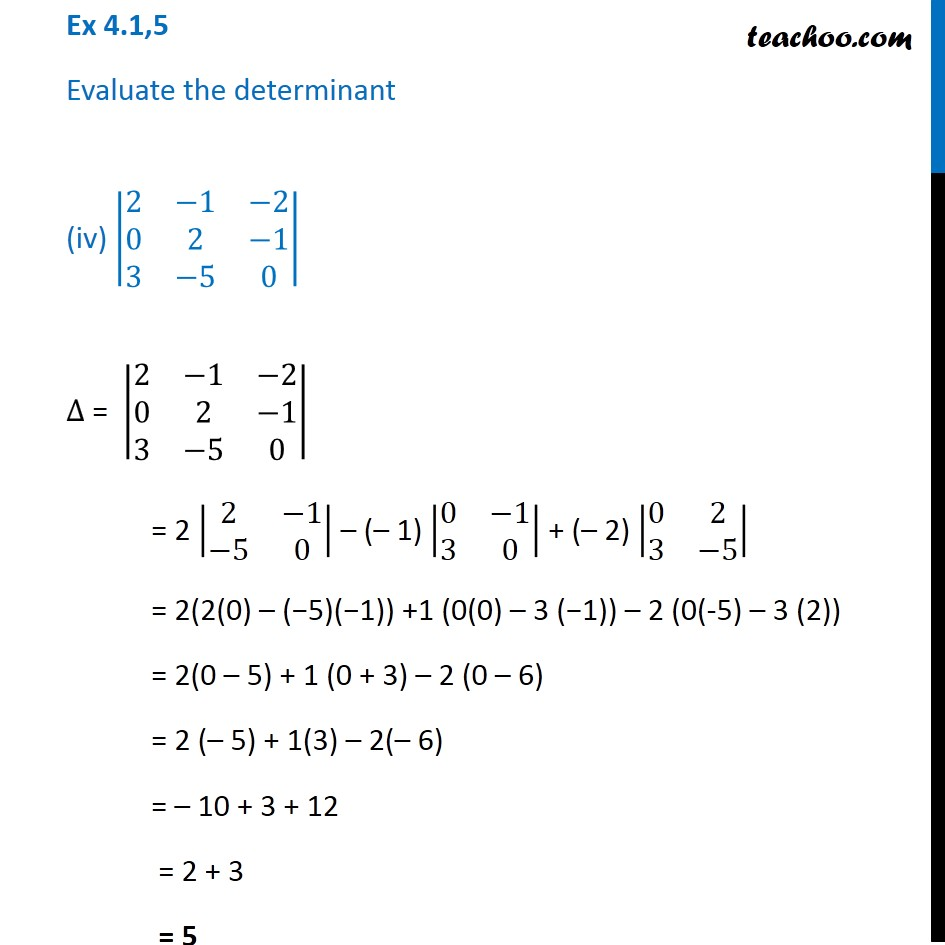Subscribe to our Youtube Channel - https://you.tube/teachoo

1. Chapter 4 Class 12 Determinants
2. Serial order wise
3. Ex 4.1

Transcript

Ex 4.1, 5 Evaluate the determinant (i) |■8(3&−1&−2@0&0&−1@3&−5&0)| |■8(3&−1&−2@0&0&−1@3&−5&0)| = 3 |■8(0&−1@−5&0)| – (–1) |■8(0&−1@3&0)| +(–2) |■8(0&0@3&−5)| = 3 (0(0) – (–5) (–1) ) + 1 (0(0) – 3(– 1)) – 2 (0(–5) – 3(0)) = 3(0 – 5) + 1 (0 + 3) – 2 (0 – 0) = 3(–5) + 1 (3) + 0 = –15 + 3 = – 12 Ex 4.1, 5 Evaluate the determinant (ii) |■8(3&−4&5@1&1&−2@2&3&1)| ∆ = |■8(3&−4&5@1&1&−2@2&3&1)| = 3 |■8(1&−2@3&1)| – (– 4)|■8(1&−2@2&1)| + 5 |■8(1&1@2&3)| = 3(1(1) –3(−2)) + 4 (1(1) –(2)(−2)) + 5 (1 (3) –2 (1)) = 3 (1 + 6) + 4 (1 + 4) + 5 (3 – 2) = 3(7) + 4 (5) + 5(1) = 21 + 20 + 5 = 46 Ex 4.1,5 Evaluate the determinants (iii) |■8(0&1&2@−1&0&−3@−2&3&0)| |■8(0&1&2@−1&0&−3@−2&3&0)| = 0 |■8(0&−3@3&0)| − 1 |■8(−1&−3@−2&0)| + 2 |■8(−1&0@−2&3)| = 0 (0(0)−3(−3))−1(−1(0)−(−2) (−3))+2 (−1(3)−(−2)0) = 0 (0 + 9) – 1 (0 – 6) +2 (– 3 – 0) = 0 – 1 (0 – 6) + 2 (−3 − 0) = –1(–6) + 2 (−3) = 6 – 6 = 0 Ex 4.1,5 Evaluate the determinant (iv) |■8(2&−1&−2@0&2&−1@3&−5&0)| ∆ = |■8(2&−1&−2@0&2&−1@3&−5&0)| = 2 |■8(2&−1@−5&0)| – (– 1) |■8(0&−1@3&0)| + (– 2) |■8(0&2@3&−5)| = 2(2(0) – (−5)(−1)) +1 (0(0) – 3 (−1)) – 2 (0(-5) – 3 (2)) = 2(0 – 5) + 1 (0 + 3) – 2 (0 – 6) = 2 (– 5) + 1(3) – 2(– 6) = – 10 + 3 + 12 = 2 + 3 = 5

Ex 4.1